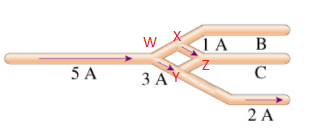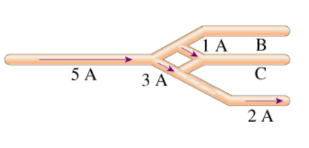# Problem: The currents through several segments of a wire object are shown in (Figure 1).Part A. What is the magnitude of the current IB in segment B?Part B. What is the direction of the current IB in segment B?

###### FREE Expert Solution(1)

Applying Kirchoff's junction rule at junction W, we have:

5 A = 3 A + iWX

iWX = 5 A - 3 A = 2 A

87% (499 ratings)###### Problem Details

The currents through several segments of a wire object are shown in (Figure 1).Part A. What is the magnitude of the current IB in segment B?

Part B. What is the direction of the current IB in segment B?